« Vrati se

IMO Shortlist 1995 problem G8

Suppose that$ABCD$ is a cyclic quadrilateral. Let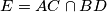$E = AC\cap BD$ and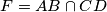$F = AB\cap CD$. Denote by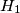$H_{1}$ and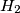$H_{2}$ the orthocenters of triangles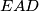$EAD$ and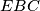$EBC$, respectively. Prove that the points$F$,$H_{1}$,$H_{2}$ are collinear.

Original formulation:

Let$ABC$ be a triangle. A circle passing through$B$ and$C$ intersects the sides$AB$ and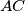$AC$ again at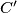$C'$ and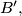$B',$ respectively. Prove that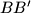$BB'$,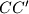$CC'$ and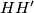$HH'$ are concurrent, where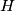$H$ and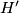$H'$ are the orthocentres of triangles$ABC$ and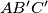$AB'C'$ respectively.